Next: Angular Momentum Operators Up: Multipole Expansion Previous: Introduction

# Multipole Expansion of Scalar Wave Equation

Before considering the vector wave equation, let us consider the somewhat simpler scalar wave equation. A scalar field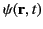satisfying the homogeneous wave equation,(1409)

can be Fourier analyzed in time,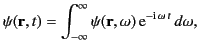(1410)

with each Fourier harmonic satisfying the homogeneous Helmholtz wave equation,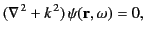(1411)

where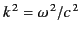. We can write the Helmholtz equation in terms of spherical coordinates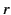,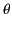,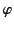: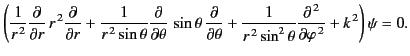(1412)

As is well known, it is possible to solve this equation via separation of variables to give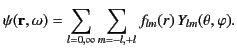(1413)

Here, we restrict our attention to physical solutions that are well-behaved in the angular variablesand. The spherical harmonics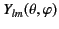satisfy the following equations: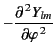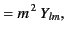(1414)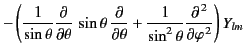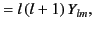(1415)

where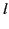is a non-negative integer, and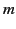is an integer that satisfies the inequality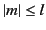. The radial functions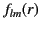satisfy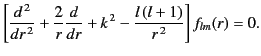(1416)

With the substitution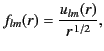(1417)

Equation (1418) is transformed into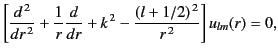(1418)

which is a type of Bessel equation of half-integer order,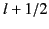. Thus, we can write the solution foras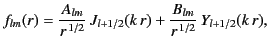(1419)

where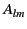and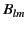are arbitrary constants. The half-integer order Bessel functions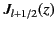and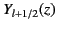have analogous properties to the integer order Bessel functions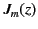and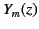. In particular, theare well behaved in the limit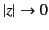, whereas theare badly behaved.

It is convenient to define the spherical Bessel functions,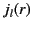and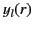, where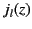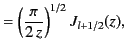(1420)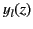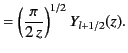(1421)

It is also convenient to define the spherical Hankel functions,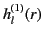and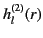, where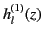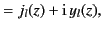(1422)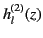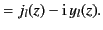(1423)

Assuming that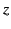is real,is the complex conjugate of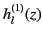. It turns out that the spherical Bessel functions can be expressed in the closed form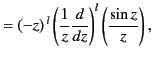(1424)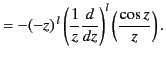(1425)

In the limit of small argument,(1426)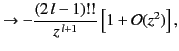(1427)

where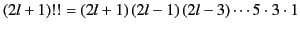. In the limit of large argument,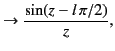(1428)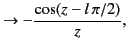(1429)

which implies that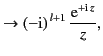(1430)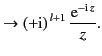(1431)

It follows, from the above discussion, that the radial functions, specified in Equation (1421), can also be written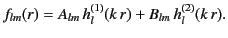(1432)

Hence, the general solution of the homogeneous Helmholtz equation, (1413), takes the form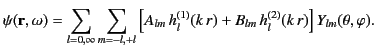(1433)

Moreover, it is clear from Equations (1412) and (1432)-(1433) that, at large, the terms involving the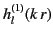Hankel functions correspond to outgoing radial waves, whereas those involving the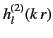functions correspond to incoming radial waves.Next: Angular Momentum Operators Up: Multipole Expansion Previous: Introduction
Richard Fitzpatrick 2014-06-27